V.5 No 2 11 On reality of black holesWhile in reality these solutions lead to absurdity. To show it, wrote the metric applied by Oppenheimer and Snyder to the inner space of star after equalising to zero the pressure and tensors of energy-momentum. They wrote this metric so: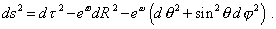(55)
 As we see, in this metric g44 = 1 , this means an homogeneous flow of time dependent of no gravity potentials. The tensors g11 , g22 and g33  have an exponential appearance; on one hand it looks already usual for relativistic metrics, but on the other it raises big questions. First will be the question of the reason of namely such representation of tensor. Both in Schwarzschild's model and in Tolman's model, which Oppenheimer and Snyder took as the basis, the resulting appearance of these tensors in the solution lose their exponentiality and the authors themselves write down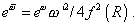(56)
 The secret is simply explainable. It is known, in integrating and differentiating, exponential function passes into itself. But on the other hand it means that to facilitate, for example, the integrating, we may substitute the inconvenient terms of differential equations by convenient functions. If we substitute the values of (56) to (55) and try to yield the same integrals of Einsteinian field equations, we simply will not be able. Should such operation be admissible in mathematical formalism, it would be very easy to substitute inconvenient under-integral expression, e.g., in elliptic integrals by convenient exponential functions and to easily yield some solutions. But will they be correct? Could we, differentiating, yield the initial under-integral expressions? Of course, no. Nothing of surprise that with such approach, relativists yield any solutions they wish from the initial equations which through correct operations give basically other results, as we showed it in a typical example, when studying Schwarzschild's derivation. Well, of which solution in relativistic case are we speaking? Of which mathematics, its formalism, rigour, proof can we ever speak? Of course, with such 'mathematical' vision of the world, there remains only a set of symbols and operations that are shuffled in the sequence and by the rules that follow not from the laws of mathematics but from the authors' wish. But the results are according to the methods. In particular, we saw above that the metric which Oppenheimer and Snyder presented was homogeneous in the local time. but in accordance with the solution, this already is not so. "When t (in Schwarzschild's metric for the outer area - Authors) tends to infinity at fixed R, the valuetends to a finite limiting value which is the more the more R. is. After this moment0  the observer who accompanies the substance already cannot send a light signal from the star - the cone within which the leaving signal is encapsulated will fully collapse" [28, p. 360]. Thus, in the initial metric (55) the time tensor is homogeneous and constant, but in the solution it must collapse at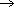0 . Moreover, "if the initial density of star was 1 g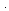cm-3 and its mass was 1033 g (approximately the mass of Sun - Authors), the duration0 is approximately one day round" [28, p. 360]. This is very easy to check, placing the observer at some as close as possible fixed distance from the Sun surface. Maybe, the Sun still is not 'burnt out', so it does not collapse? Why not? If in the metric it is possible so easily to make zero all tensors of energy-momentum, as well as the pressure in the gravitating material body, there can be no difficulty with the choice of metric in which all thermodynamic potentials become zero. And on the other hand, it can look like the calculation methods for black holes which we analysed here are some particularities and there can be found some other ways to derive black holes. To a great regret of relativists, we have to give a negative answer. The conditions of zero pressure in the gravitating body, and especially neglect of dependence of this internal pressure on the degree of compression of the gravitating mass, are an important and inalienable conditions of black hole modelling. In a synchronous inertial reference frame, "'trial' particles being at rest relatively the reference frame are the particles freely moving in this field" [23, p. 394]. It to become realisable, these particles have to meet on their way no resistance either viscous friction and to be not reflected from other particles. This last is possible exceptionally at the conditions that have been put into the model of collapsing dust-like sphere, in Schwarzschild's model, in Oppenheimer's model. Only in this case "to the given values of r the world lines R - c= const   (inclined direct lines in the diagram) correspond. While the world lines of particles being at rest relatively the reference frame are shown in this diagram as vertical direct lines; moving along them, the particles during a finite interval of their own time 'fall' onto the centre of field (r = 0) that is the point of true singularity of metric" [23, p. 394]. And the inverse conclusion follows from this analysis. If we have no right to turn to zero the tensor of energy-momentum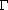and if we may not neglect the internal processes in a celestial body that cause the counter-pressure and/or heating of this body, the relativistic formalism of black holes, with all its shuffling of symbols, all artificially introduced metrics, incorrect mathematics, far-fetched geometric imaged of physical processes, appears unworkable and fully loses its validity both in the statement of problem and in solutions. Nothing of surprise that with all attempts of relativistic astrophysicists to find in real nature some observable corroboration to their 'mathematical' view, they confine themselves to cheap one-day sensations that are dispelled with the first analysis of obtained material.

Contents: / 1 / 2 / 3 / 4 / 5 / 6 / 7 / 8 / 9 / 10 / 11 / 12 / 13 / 14 / 15 / 16 / 17 /